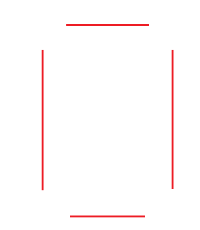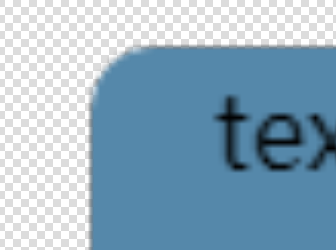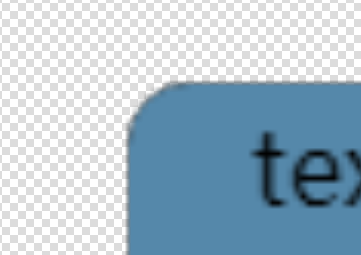# 详解微信小程序canvas圆角矩形的绘制的方法

更新时间：2018年08月22日 13:45:41   作者：清夜我要评论`clip`

`save``restore`

```/**
*
* @param {CanvasContext} ctx canvas上下文
* @param {number} x 圆角矩形选区的左上角 x坐标
* @param {number} y 圆角矩形选区的左上角 y坐标
* @param {number} w 圆角矩形选区的宽度
* @param {number} h 圆角矩形选区的高度
* @param {number} r 圆角的半径
*/
function roundRect(ctx, x, y, w, h, r) {
// 开始绘制
ctx.beginPath()
// 因为边缘描边存在锯齿，最好指定使用 transparent 填充
// 这里是使用 fill 还是 stroke都可以，二选一即可
ctx.setFillStyle('transparent')
// ctx.setStrokeStyle('transparent')
// 左上角
ctx.arc(x + r, y + r, r, Math.PI, Math.PI * 1.5)

// border-top
ctx.moveTo(x + r, y)
ctx.lineTo(x + w - r, y)
ctx.lineTo(x + w, y + r)
// 右上角
ctx.arc(x + w - r, y + r, r, Math.PI * 1.5, Math.PI * 2)

// border-right
ctx.lineTo(x + w, y + h - r)
ctx.lineTo(x + w - r, y + h)
// 右下角
ctx.arc(x + w - r, y + h - r, r, 0, Math.PI * 0.5)

// border-bottom
ctx.lineTo(x + r, y + h)
ctx.lineTo(x, y + h - r)
// 左下角
ctx.arc(x + r, y + h - r, r, Math.PI * 0.5, Math.PI)

// border-left
ctx.lineTo(x, y + r)
ctx.lineTo(x + r, y)

// 这里是使用 fill 还是 stroke都可以，二选一即可，但是需要与上面对应
ctx.fill()
// ctx.stroke()
ctx.closePath()
// 剪切
ctx.clip()
}```

ps：微信小程序canvas把正方形图片绘制成圆形

`<canvas style="width: 400px; height: 400px;border:1px solid red" canvas-id="firstCanvas"></canvas>`
```//index.js
Page({
data: {
image: {
src: "/1.png",
width: 200,
heigth: 200
}
},
onLoad: function () {
let that = this;
var contex = wx.createCanvasContext('firstCanvas')
contex.save(); // 先保存状态 已便于画完圆再用
contex.beginPath(); //开始绘制
//先画个圆
contex.arc(100, 100, 100, 0, Math.PI * 2, false);
contex.clip();//画了圆 再剪切 原始画布中剪切任意形状和尺寸。一旦剪切了某个区域，则所有之后的绘图都会被限制在被剪切的区域内
contex.drawImage(that.data.image.src, 0, 0, that.data.image.width, that.data.image.heigth); // 推进去图片
contex.restore(); //恢复之前保存的绘图上下文 恢复之前保存的绘图上下午即状态 可以继续绘制
contex.draw();
}
})

```